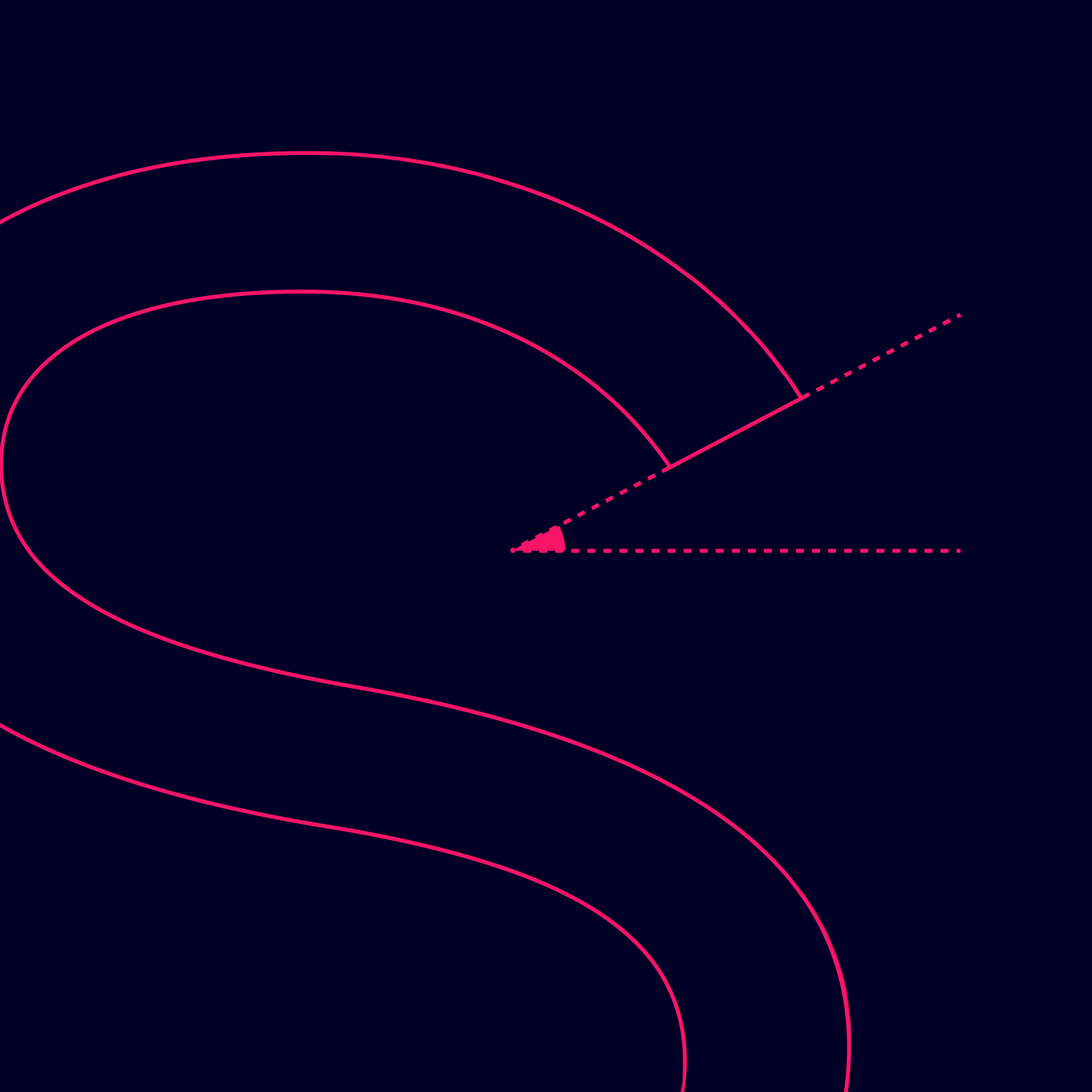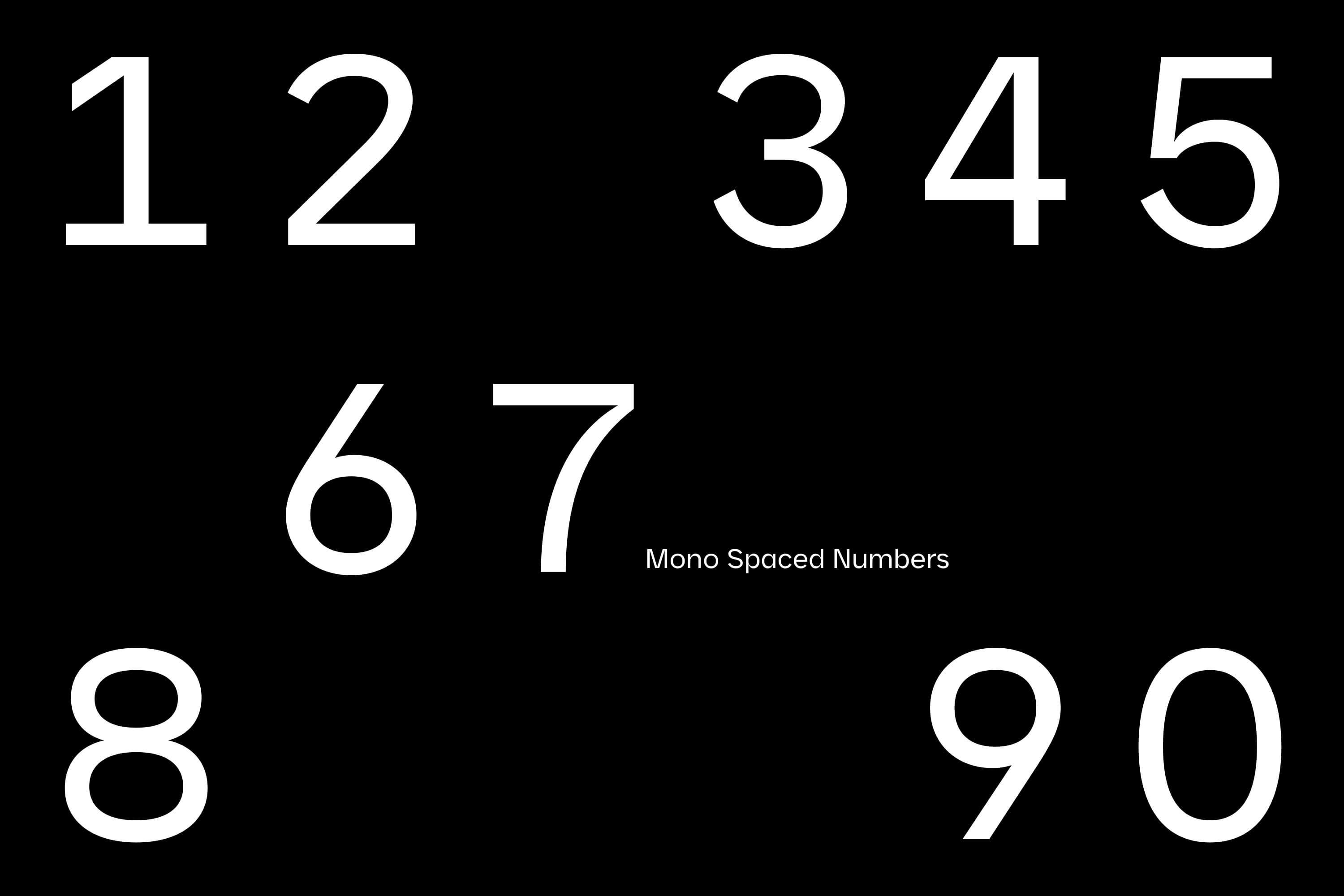# GD Ohio

GD Ohio is a font that is versatile and designed for optimal visibility from a distance. It is suitable for both bold, heavy statements and regular information in lighter weights. The font is highly adaptable, ranging from calm and subdued to loud and energetic, making it perfect for visual identities used in high-speed highways and small, static assets. As a variable font, GD Ohio can be adjusted to take on different shapes and sizes, making it ideal for a wide range of applications, including logos, signage, text-heavy documents, and webpages. Its broad range of weights, widths, and styles makes it perfect for creating a unique and eye-catching visual brand identity.

In December 2022, it was implemented for Billo in both their app and website with minor adjustments and renamed as Billo Sans.

Hej

Styles

Overview

SemiExpanded Regular

UltraBold

Expanded Regular

Expanded UltraBold

Medium

Regular

Bold

Expanded Bold

Expanded Medium

Expanded SemiBold

SemiBold

SemiExpanded Bold

SemiExpanded Medium

SemiExpanded SemiBold

SemiExpanded UltraBoldPreview styles

A

A

B

C

D

E

F

G

H

I

J

K

L

M

N

O

P

Q

R

S

T

U

V

W

X

Y

Z

Å

Ä

Ö

a

b

c

d

e

f

g

h

i

j

k

l

m

n

o

p

q

r

s

y

u

v

w

x

y

z

á

â

å

ä

ö

.

,

:

;

!

¡

?

#

/

\

-

_

(

)

{

}

[

]

0

1

2

3

4

5

6

7

9

8

A

A

B

C

D

E

F

G

H

I

J

K

L

M

N

O

P

Q

R

S

T

U

V

W

X

Y

Z

Å

Ä

Ö

a

b

c

d

e

f

g

h

i

j

k

l

m

n

o

p

q

r

s

y

u

v

w

x

y

z

á

â

å

ä

ö

.

,

:

;

!

¡

?

#

/

\

-

_

(

)

{

}

[

]

0

1

2

3

4

5

6

7

9

8

A

A

B

C

D

E

F

G

H

I

J

K

L

M

N

O

P

Q

R

S

T

U

V

W

X

Y

Z

Å

Ä

Ö

a

b

c

d

e

f

g

h

i

j

k

l

m

n

o

p

q

r

s

y

u

v

w

x

y

z

á

â

å

ä

ö

.

,

:

;

!

¡

?

#

/

\

-

_

(

)

{

}

[

]

0

1

2

3

4

5

6

7

9

8

A

A

B

C

D

E

F

G

H

I

J

K

L

M

N

O

P

Q

R

S

T

U

V

W

X

Y

Z

Å

Ä

Ö

a

b

c

d

e

f

g

h

i

j

k

l

m

n

o

p

q

r

s

y

u

v

w

x

y

z

á

â

å

ä

ö

.

,

:

;

!

¡

?

#

/

\

-

_

(

)

{

}

[

]

0

1

2

3

4

5

6

7

9

8

A

A

B

C

D

E

F

G

H

I

J

K

L

M

N

O

P

Q

R

S

T

U

V

W

X

Y

Z

Å

Ä

Ö

a

b

c

d

e

f

g

h

i

j

k

l

m

n

o

p

q

r

s

y

u

v

w

x

y

z

á

â

å

ä

ö

.

,

:

;

!

¡

?

#

/

\

-

_

(

)

{

}

[

]

0

1

2

3

4

5

6

7

9

8

A

A

B

C

D

E

F

G

H

I

J

K

L

M

N

O

P

Q

R

S

T

U

V

W

X

Y

Z

Å

Ä

Ö

a

b

c

d

e

f

g

h

i

j

k

l

m

n

o

p

q

r

s

y

u

v

w

x

y

z

á

â

å

ä

ö

.

,

:

;

!

¡

?

#

/

\

-

_

(

)

{

}

[

]

0

1

2

3

4

5

6

7

9

8

A

A

B

C

D

E

F

G

H

I

J

K

L

M

N

O

P

Q

R

S

T

U

V

W

X

Y

Z

Å

Ä

Ö

a

b

c

d

e

f

g

h

i

j

k

l

m

n

o

p

q

r

s

y

u

v

w

x

y

z

á

â

å

ä

ö

.

,

:

;

!

¡

?

#

/

\

-

_

(

)

{

}

[

]

0

1

2

3

4

5

6

7

9

8

A

A

B

C

D

E

F

G

H

I

J

K

L

M

N

O

P

Q

R

S

T

U

V

W

X

Y

Z

Å

Ä

Ö

a

b

c

d

e

f

g

h

i

j

k

l

m

n

o

p

q

r

s

y

u

v

w

x

y

z

á

â

å

ä

ö

.

,

:

;

!

¡

?

#

/

\

-

_

(

)

{

}

[

]

0

1

2

3

4

5

6

7

9

8

A

A

B

C

D

E

F

G

H

I

J

K

L

M

N

O

P

Q

R

S

T

U

V

W

X

Y

Z

Å

Ä

Ö

a

b

c

d

e

f

g

h

i

j

k

l

m

n

o

p

q

r

s

y

u

v

w

x

y

z

á

â

å

ä

ö

.

,

:

;

!

¡

?

#

/

\

-

_

(

)

{

}

[

]

0

1

2

3

4

5

6

7

9

8

A

A

B

C

D

E

F

G

H

I

J

K

L

M

N

O

P

Q

R

S

T

U

V

W

X

Y

Z

Å

Ä

Ö

a

b

c

d

e

f

g

h

i

j

k

l

m

n

o

p

q

r

s

y

u

v

w

x

y

z

á

â

å

ä

ö

.

,

:

;

!

¡

?

#

/

\

-

_

(

)

{

}

[

]

0

1

2

3

4

5

6

7

9

8

A

A

B

C

D

E

F

G

H

I

J

K

L

M

N

O

P

Q

R

S

T

U

V

W

X

Y

Z

Å

Ä

Ö

a

b

c

d

e

f

g

h

i

j

k

l

m

n

o

p

q

r

s

y

u

v

w

x

y

z

á

â

å

ä

ö

.

,

:

;

!

¡

?

#

/

\

-

_

(

)

{

}

[

]

0

1

2

3

4

5

6

7

9

8

A

A

B

C

D

E

F

G

H

I

J

K

L

M

N

O

P

Q

R

S

T

U

V

W

X

Y

Z

Å

Ä

Ö

a

b

c

d

e

f

g

h

i

j

k

l

m

n

o

p

q

r

s

y

u

v

w

x

y

z

á

â

å

ä

ö

.

,

:

;

!

¡

?

#

/

\

-

_

(

)

{

}

[

]

0

1

2

3

4

5

6

7

9

8

A

A

B

C

D

E

F

G

H

I

J

K

L

M

N

O

P

Q

R

S

T

U

V

W

X

Y

Z

Å

Ä

Ö

a

b

c

d

e

f

g

h

i

j

k

l

m

n

o

p

q

r

s

y

u

v

w

x

y

z

á

â

å

ä

ö

.

,

:

;

!

¡

?

#

/

\

-

_

(

)

{

}

[

]

0

1

2

3

4

5

6

7

9

8

A

A

B

C

D

E

F

G

H

I

J

K

L

M

N

O

P

Q

R

S

T

U

V

W

X

Y

Z

Å

Ä

Ö

a

b

c

d

e

f

g

h

i

j

k

l

m

n

o

p

q

r

s

y

u

v

w

x

y

z

á

â

å

ä

ö

.

,

:

;

!

¡

?

#

/

\

-

_

(

)

{

}

[

]

0

1

2

3

4

5

6

7

9

8

A

A

B

C

D

E

F

G

H

I

J

K

L

M

N

O

P

Q

R

S

T

U

V

W

X

Y

Z

Å

Ä

Ö

a

b

c

d

e

f

g

h

i

j

k

l

m

n

o

p

q

r

s

y

u

v

w

x

y

z

á

â

å

ä

ö

.

,

:

;

!

¡

?

#

/

\

-

_

(

)

{

}

[

]

0

1

2

3

4

5

6

7

9

8

Fonts in use

Overview